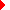﻿ System Variables
SilverScreen Solid Modeler

# System Variables# System Variables# System Variables

SilverScreen system variables are named storage objects that are global to the SilverScreen system. They are useful for communicating between programs.  They may also be used to retrieve the results of certain SilverScreen commands (see below).

Three types of system variables are allowed: value, text and XYZ. Value variables are used to hold a single floating point number (double). Text variables are used to hold a text string (char ). XYZ variables are used to hold the location of a point in 3D space. System variables may be accessed via the load_variable function or the sysvar functions.

Assigning and Unassigning

In SilverScreen, system variables are created, destroyed and assigned by using VARIABLE commands.They can be assigned a value by the VARIABLE ASSIGN script command. The command has three forms, each corresponding to a data type that can be associated with a system variable:

variable assign <name> value <value>

variable assign <name> text <string>

variable assign <name> xyz <xyz location>Groups of variables can be assigned values by means of a VARIABLE LOAD command. This command is related to the VARIABLE SAVE command. The latter command saves all currently active variables in disk file with extension .VAR. These variables can then be reloaded with the VARIABLE LOAD command. See the SilverScreen manual for further details on these commands.Groups of variables may also be assigned values with the VARIABLE V-EDIT command. This command is essentially a variant of the VARIABLE LOAD command. For further information on this topic, see the SilverScreen manual and the section on the V-EDIT command.Variables can be assigned values as a result of a MEASURE command. There are roughly a dozen variables that are set by this command to reflect the result of a measurement. A listing of these variables appears at the end of this section.Variables retain their values until they are unassigned with the VARIABLE UNASSIGN command, or until the CLEAR command is issued.

MEASURE Command

A number of SilverScreen commands produce results that are assigned to various system variables. The commands that currently produce sys­tem variable results all belong in the GEOMETRY MEASURE family.

The following table describes the variable created, the SilverScreen command that creates it and the data type of the variable created (the GEOMETRY prefix is not included in the command description):

 Variable SilverScreen Command Data Type \$angle MEASURE ANGLE value \$area MEASURE AREA value \$centroid MEASURE CENTROID XYZ \$dimx MEASURE DIMENSIONS value \$dimy MEASURE DIMENSIONS value \$dimz MEASURE DIMENSIONS value \$distance MEASURE DISTANCE value \$length MEASURE LENGTH value \$ixx MEASURE MOMENT CENTROID value \$ixx MEASURE MOMENT ORIGIN value \$ixy MEASURE MOMENT CENTROID value \$ixy MEASURE MOMENT ORIGIN value \$ixz MEASURE MOMENT CENTROID value \$ixz MEASURE MOMENT ORIGIN value \$moment MEASURE MOMENT AXIS value \$moment MEASURE MOMENT ENTITY AXIS value \$volume MEASURE VOLUME value

Here is an example where the MEASURE command is used to measure the area of a polygon:

 C / C++ Code double area;  ...  ss_command ( "measure area primitive polygon \\arm\\bolt4.26" );  sysvar_value (“\$area”,&area);  ss_command ( "note The area of \\arm\\bolt4.26 is %f", area );